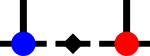SyTen## ◆ operator==() [7/7]

template<Rank rank>
 bool syten::operator== ( Tensor< rank > const & a, Tensor< rank > const & b )
inline

Returns true if a and b are identical up to error sqrt(N)·ε where N is the average of the number of dense entries in a and b and ε is SYTEN_SMALL_THRESHOLD.

For big tensors, this is a fairly relaxed measure.Here is the call graph for this function: